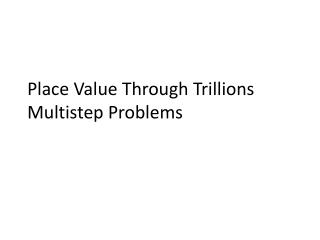# Place Value Through Trillions Multistep Problems - PowerPoint PPT PresentationDownload PresentationPlace Value Through Trillions Multistep Problems

Place Value Through Trillions Multistep ProblemsDownload Presentation## Place Value Through Trillions Multistep Problems

- - - - - - - - - - - - - - - - - - - - - - - - - - - E N D - - - - - - - - - - - - - - - - - - - - - - - - - - -
##### Presentation Transcript

1. Place Value Through TrillionsMultistep Problems

2. Place Value • Commas

3. Use digits to write the number one trillion, two hundred fifty billion.

4. Multistep Problems Operations of Arithmetic

5. What is the difference between the product of 6 and 4 and the sum of 6 and 4?

6. Which digit is in the millions place in 123,456,789?

7. What is the place value of the 1 in 12,453,000,000?

8. When the product of 6 and 4 is divided by the difference of 6 and 4, what is the quotient?

9. In the number 123,456,789,000, which digit is in the ten-millions place?